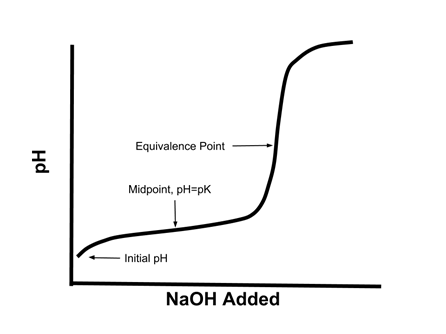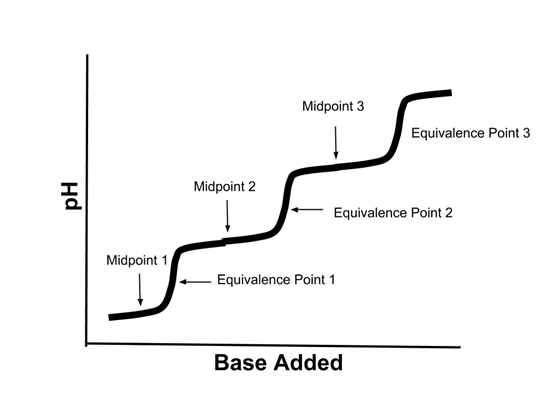# Enduring Understanding 6.C: Titration Curves

• When a solution of a weak acid HA is titrated with a strong base like NaOH, the titration curve (graph of pH vs. NaOH added) looks like this:
•• When one half an equivalent of NaOH is added, the pH is equal to the pKa of the weak acid.
• At the half-equivalence point, half the acid has reacted to form the conjugate base, so [HA] = [A-]
• According to the Henderson Hasselbach equation:
• pH = pKa + log [A-]/[HA]
• Since log [A-]/[HA] = 0, pH = pKa
• At the equivalence point, all the acid has reacted with the base, so the solution is effectively a solution of NaA.
• Sample Question 1: What is the pH of 50 mL of a 0.1M CH3COOH solution titrated to its equivalence point with 50 mL of an 0.1M NaOH? pKa of acetate = 4.75

• All the CH3COOH has been converted to CH3COO-
• The concentration of CH3COO- is (0.05L x 0.1 mol/L) / 0.10 L or 0.05 mol/L
• The CH3COO- will react with the water to form hydroxide:
• CH3COO- + H2O → OH- + CH3COOH
• We can use the Kb of acetate to calculate the concentration of hydroxide present.
• The pKb for acetate is (14-pKa) or 9.25, so the Kb is 10-9.25 or 5.6 x 10-10
• [OH-] □ [CH3COOH]
• Kb = 5.6 x 10-10 = [CH3COOH] [OH-] / [CH3COO-] = [OH-]2 / 0.05 mol/L
• [OH-]2 = 2.8 x 10-11, so [OH-] = 5.3 x 10-6
• pOH= -log [OH-] = 5.27
• pH = (14-pOH) = 8.73
• Titration curves of polyprotic acids are more complex. Here is the titration curve of a triprotic acid:
•• If the starting acid was H3PO4, then:
• Midpoint 1 would represent the point where [H3PO4]=[H2PO4-]
• Midpoint 2 would represent the point where [H2PO4-]=[HPO42-]
• Midpoint 3 would represent the point where [HPO42-]=[PO43-]
• Equivalence point 1 represent the point where the [H3PO4] was completely reacted with the base.
• Equivalence point 2 represent the point where the [H2PO4-] was completely reacted with the base.
• Equivalence point 3 represent the point where the [HPO42-] was completely reacted with the base.

 Related Links: Chemistry Chemistry Quizzes AP Chemistry Notes Le Chatelier's Principle

To link to this Titration Curves page, copy the following code to your site: